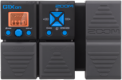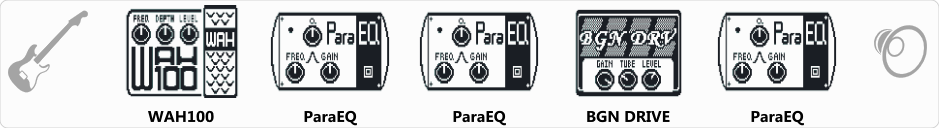# KL MoneyDIRE+

Discussion in 'Zoom G1on/G1Xon' started by Kovács László, Jun 11, 2018.

1. KL MoneyDIRE+Device: Zoom G1Xon
Firmware: 1.21

Name on device: MoneyDIRE+
Optimized for: Phones/Speaker

Effects chain:Use pedal for output volume!

Effect: "WAH100" (Pedal), active - "yes"

"Freq" = 2

"Depth" = 100
"Level" = 136

Effect: "ParaEQ" (Dynamics / Filter), active - "yes"

"Freq1" = 160Hz

"Q1" = 1
"Gain1" = 9
"Freq2" = 400Hz
"Q2" = 1
"Gain2" = 2
"Level" = 100

Effect: "ParaEQ" (Dynamics / Filter), active - "yes"

"Freq1" = 1.0kHz

"Q1" = 1
"Gain1" = -6
"Freq2" = 10kHz
"Q2" = 1
"Gain2" = 7
"Level" = 100

Effect: "BGN DRIVE" (Amp simulators), active - "yes"

"Gain" = 20

"Tube" = 89
"Level" = 91
"Trebl" = 32
"Middl" = 21
"Bass" = 100
"Prese" = 41
"CAB" = BG CRUNCH 1x12
"OUT" = LINE

Effect: "ParaEQ" (Dynamics / Filter), active - "yes"

"Freq1" = 200Hz

"Q1" = 1
"Gain1" = -1
"Freq2" = 5.0kHz
"Q2" = 1
"Gain2" = 3
"Level" = 100
Patch Volume: 120

Note: This is a patch file, you will need to download and install the ToneLib software to use the patch.

#### Attached Files:

• ###### KL_MoneyDIRE.g1xon
File size:
425 bytes
Views:
351
Last edited: Sep 21, 2018
Dr.TPyTEHb and saobienorgan like this.
2. Super!!!